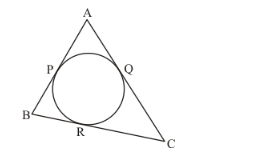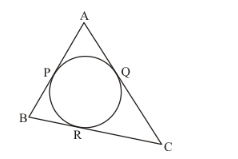# In the given figure, if AP = PB, then

Question:

In the given figure, if AP = PB, then(a) AC = AB

(b) AC = BC

(c) AQ = QC

(d) AB = BC

Solution:

The given figure is belowIt is given that,

AP = PB

We know that

PB = BR(tangents from an external point are equal)

From the above two equations, we can say that,

AP = BR …… (1)

Also,

AP = AQ(tangents from an external point are equal) …… (2)

From equations (1) and (2), we have,

AQ = BR …… (3)

Also,

QC = CR(tangents from an external point are equal) …… (4)

Adding equations (3) and (4), we get,

AQ + QC = BR + CR

By looking at the figure we can write the above equation as,

AC = BC

The correct answer is option (b).